## Insights at Disparate Scales$\phi(E,\vec{r},\hat{\Omega},T)$

$\phi(E, x, y, z, \theta, \omega, T)$$\sigma(x, i, E, T)$$\gamma(f, i)$$i$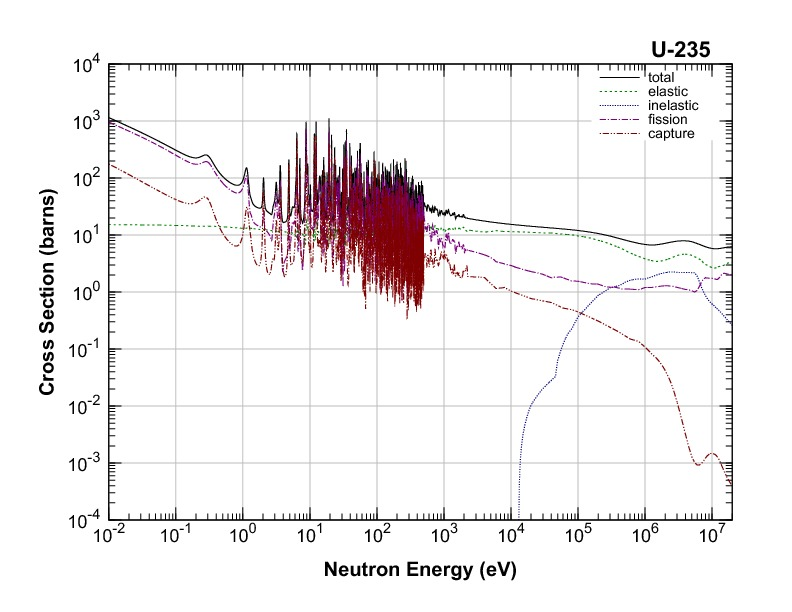$E$nuclear structure

## Evaluated Nuclear Data Sets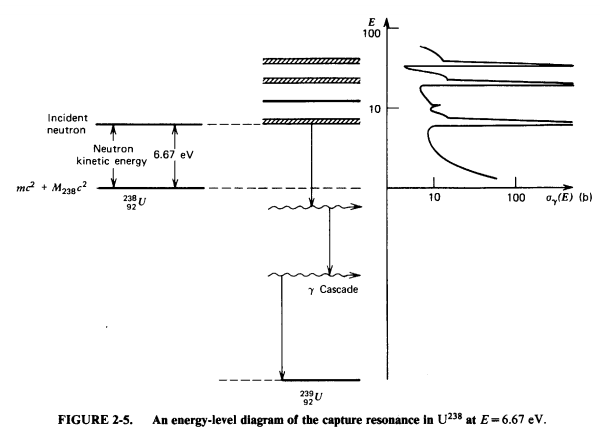$\sigma(x, i, E, T)=$ nuclear structure + experiments

## Experiments## Experiments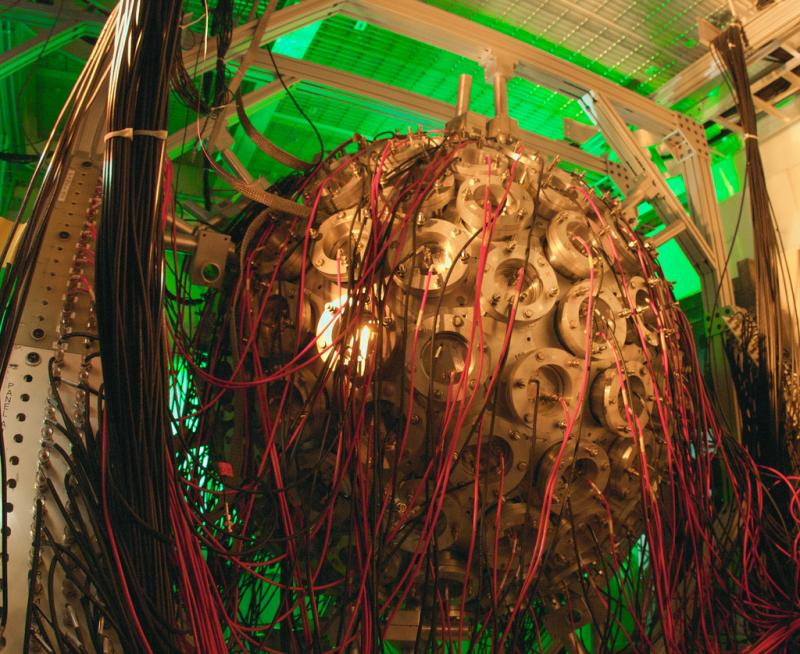## Nuclear Data is for Simulations## Simulation Methods

• Monte Carlo Methods
• Deterministic Methods
• Hybrid Methods
• Other keywords...
• lattice codes
• ray tracing algorithms
• acceleration schemes
• ...

## Application Specific Data Processing

• Energy discretization
• multigroup
• pointwise
• piecewise linear continuous
• Resonance integration
• ...

### MSBR Full Core Simulation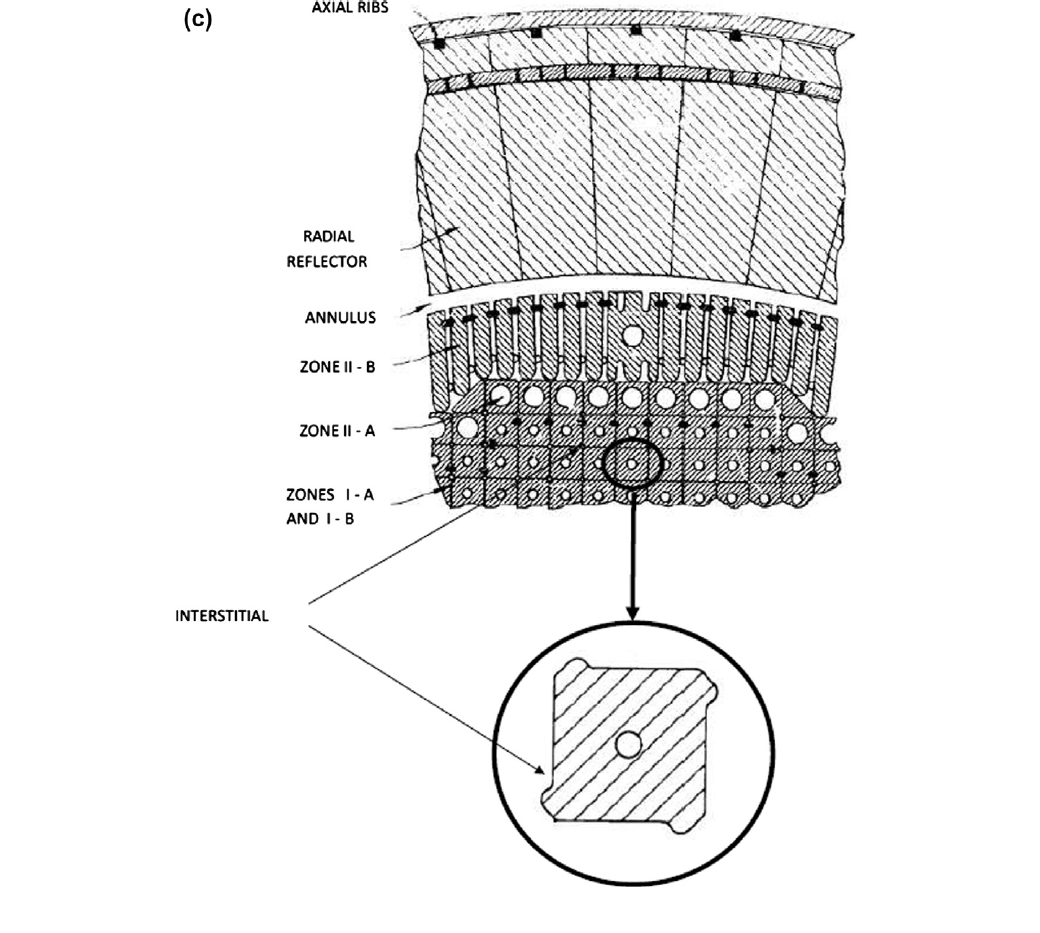(Robertson, 1979)

### MSBR Full Core Simulation

Andrei Rykhlevskii### MSBR Full Core Simulation

Andrei Rykhlevskii### MSBR Full Core Simulation

Andrei Rykhlevskii### MSBR Full Core Simulation

Andrei Rykhlevskii### MSBR Full Core Simulation

Andrei Rykhlevskii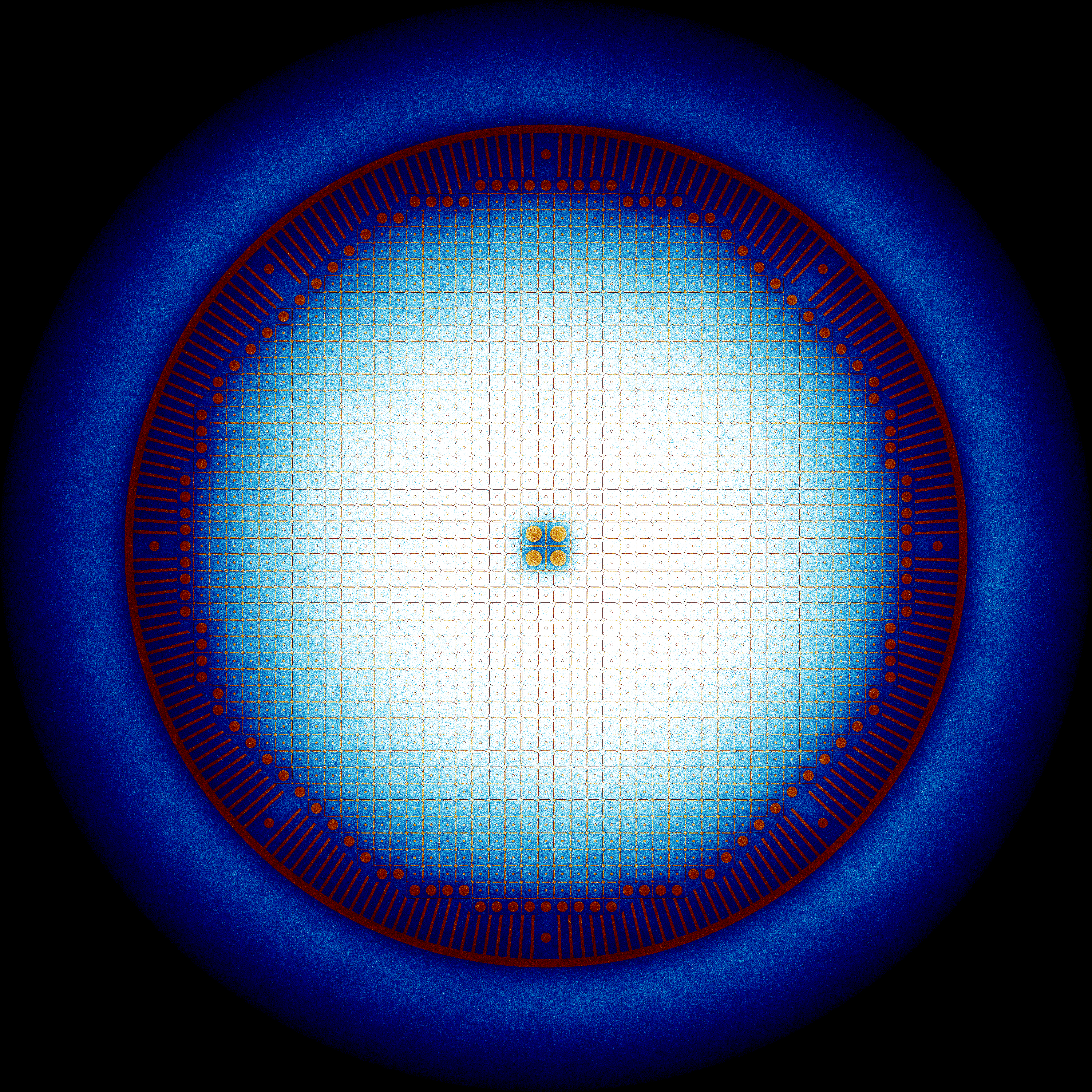### MSBR Full Core Simulation

Andrei Rykhlevskii### MSBR Full Core Simulation

Andrei Rykhlevskii### MSBR Full Core Simulation

Andrei Rykhlevskii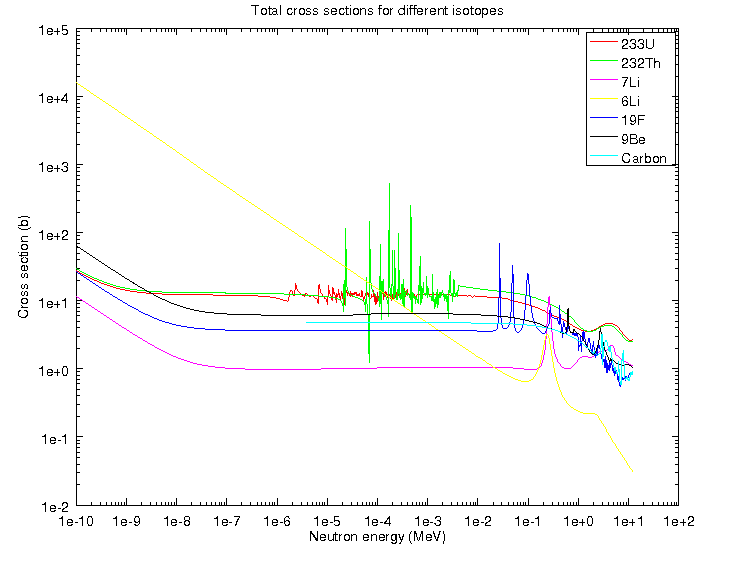### MSBR Full Core Simulation

Andrei Rykhlevskii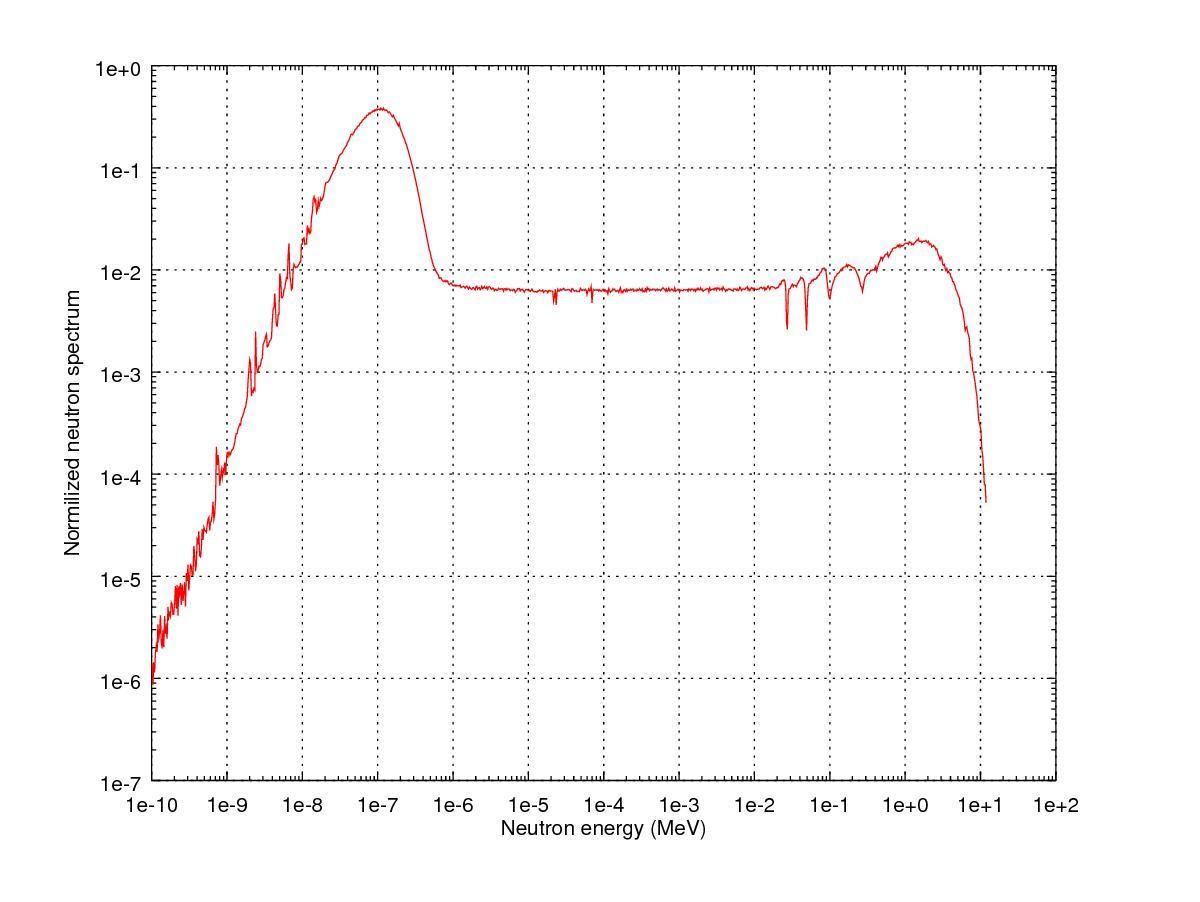## Reactor Kinetics$\sigma(E,\vec{r},\hat{\Omega},T,x,i)$$k=1$

## Reactivity

\begin{align} k &= \mbox{"neutron multiplication factor"}\\ &= \frac{\mbox{neutrons causing fission}}{\mbox{neutrons produced by fission}}\\ \rho &= \frac{k-1}{k}\\ \rho &= \mbox{reactivity}\\ \end{align}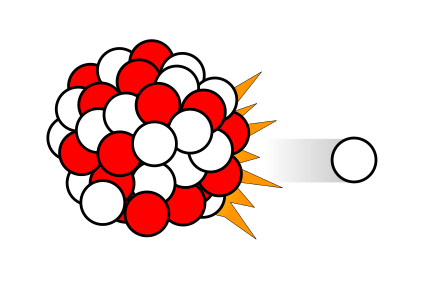$\beta_i, \lambda_{d,i}$

## Point Reactor Kinetics

\begin{align} p &= \mbox{ reactor power }\\ \rho(t,&T_{fuel},T_{cool},T_{mod}, T_{refl}) = \mbox{ reactivity}\\ \beta &= \mbox{ fraction of neutrons that are delayed}\\ \beta_j &= \mbox{ fraction of delayed neutrons from precursor group j}\\ \zeta_j &= \mbox{ concentration of precursors of group j}\\ \lambda_{d,j} &= \mbox{ decay constant of precursor group j}\\ \Lambda &= \mbox{ mean generation time }\\ \omega_k &= \mbox{ decay heat from FP group k}\\ \kappa_k &= \mbox{ heat per fission for decay FP group k}\\ \lambda_{FP,k} &= \mbox{ decay constant for decay FP group k}\\ T_i &= \mbox{ temperature of component i} \end{align}
$\frac{d}{dt}\left[ \begin{array}{c} p\\ \zeta_1\\ .\\ \zeta_j\\ .\\ \zeta_J\\ \omega_1\\ .\\ \omega_k\\ .\\ \omega_K\\ T_{i}\\ .\\ T_{I}\\ \end{array} \right] = \left[ \begin{array}{ c } \frac{\rho(t,T_{i},\cdots)-\beta}{\Lambda}p + \displaystyle\sum^{j=J}_{j=1}\lambda_{d,j}\zeta_j\\ \frac{\beta_1}{\Lambda} p - \lambda_{d,1}\zeta_1\\ .\\ \frac{\beta_j}{\Lambda}p-\lambda_{d,j}\zeta_j\\ .\\ \frac{\beta_J}{\Lambda}p-\lambda_{d,J}\zeta_J\\ \kappa_1p - \lambda_{FP,1}\omega_1\\ .\\ \kappa_kp - \lambda_{FP,k}\omega_k\\ .\\ \kappa_{k p} - \lambda_{FP,k}\omega_{k}\\ f_{i}(p, C_{p,i}, T_{i}, \cdots)\\ .\\ f_{I}(p, C_{p,I}, T_{I}, \cdots)\\ \end{array} \right]$

## Coupled Multi-Physics Analysis

Using the MOOSE framework and its Jacobian-Free Newton Krylov solver, severe accident neutronics and thermal hydraulics can be simulated beautifully for simple geometries and well studied materials. (below, INL BISON work.)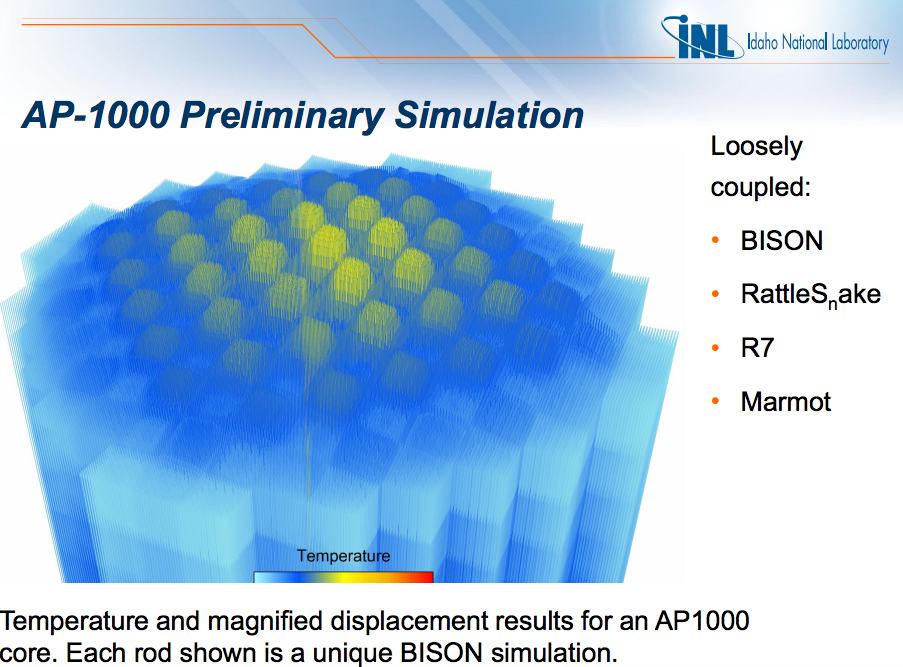## Moltres: Dr. Alexander Lindsay

### Moltres Current Capabilities

• Navier Stokes + Multi-Group Neutron Diffusion + Kinetics
• arbitrary number of neutron energy groups
• arbitrary number of delayed neutron precursor groups
• neutron power coupled to salt temperature and flow
• Precursor capability requires discontinuous galerkin upwind scheme.
• Few group constants can be generated with SCALE-NEWT or Serpent

## Insights at Disparate Scales## The Nuclear Fuel Cycle

Hundreds of discrete facilities mine, mill, convert, fabricate, transmute, recycle, and store nuclear material.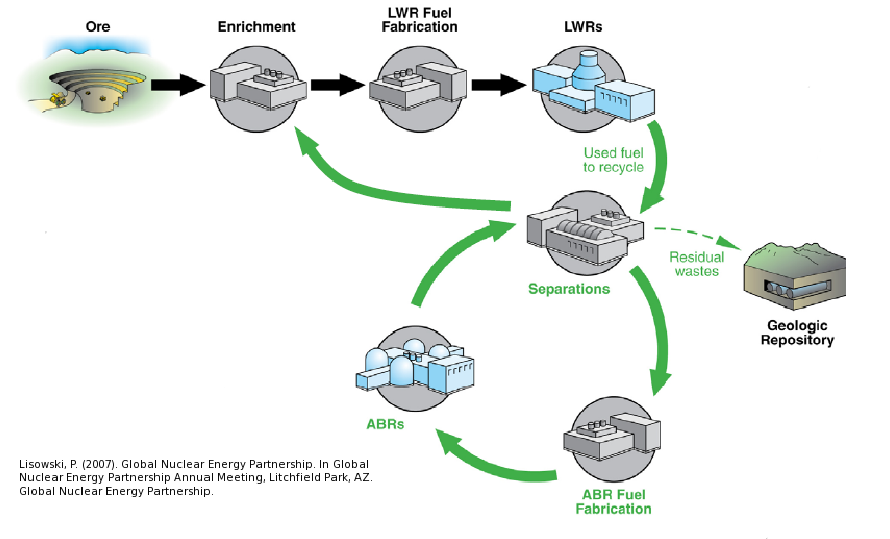## Fuel Cycle Metrics

• Mass Flow
• proliferation resistance and physical protection (PRPP) indices.
• Cost
• levelized cost of electricity,
• facility life cycle costs.
• Economics
• power production, facility deployments,
• dynamic pricing and feedback.
• Disruptions
• reliability, safety,
• system robustness.

### Current Simulators

• CAFCA (MIT)
• COSI (CEA)
• DANESS (ANL)
• DESAE (Rosatom)
• Evolcode (CIEMAT)
• FAMILY (IAEA)
• GENIUSv1 (INL)
• GENIUS v2 (UW)
• NFCSim (LANL)
• NFCSS (IAEA)
• NUWASTE (NWTRB)
• ORION (NNL)
• MARKAL (BNL)
• VISION (INL)

## State of the Art

### Performance

• Speed interactive time scales
• Fidelity: detail commensurate with existing challenges
• Detail: discrete material and agent tracking
• Regional Modeling: enabling international socio-economics

## Beyond the State of the Art

### Access

• Openness: for collaboration, validation, and code sustainability.
• Usability: for a wide range of user sophistication

### Extensibility

• Modularity: core infrastructure independent of proprietary or sensitive data and models
• Flexibility with a focus on robustness for myriad potential developer extensions.

## Extensibility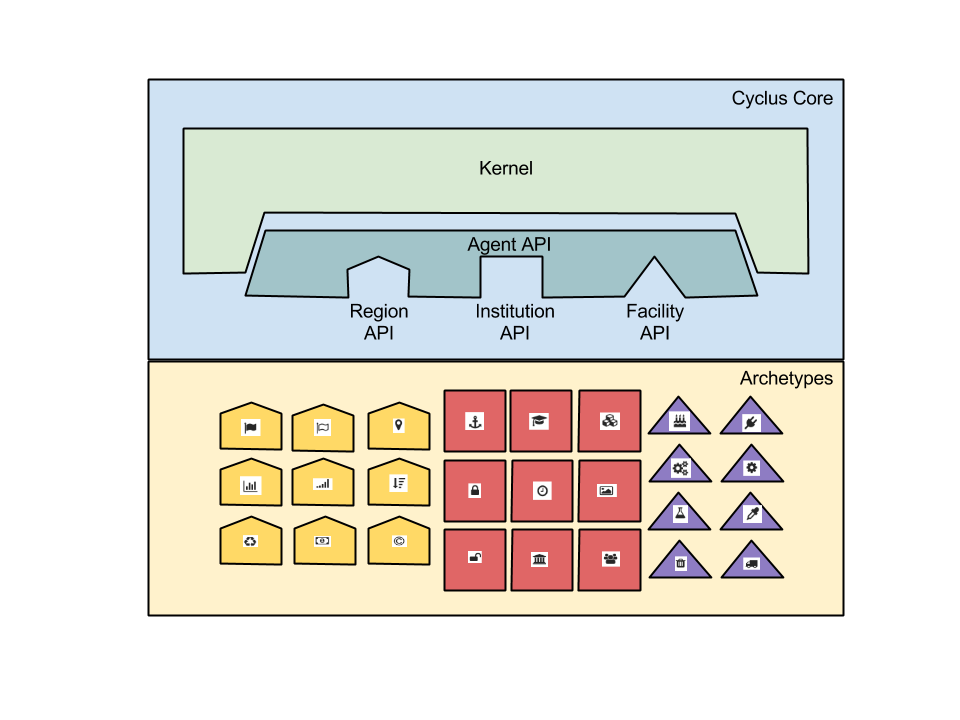## Openness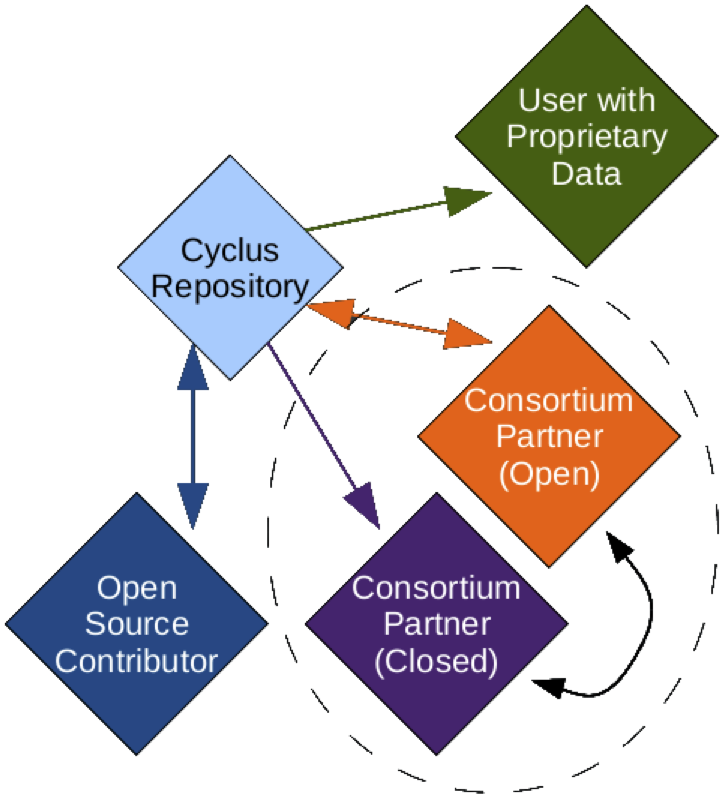## Growing Ecosystem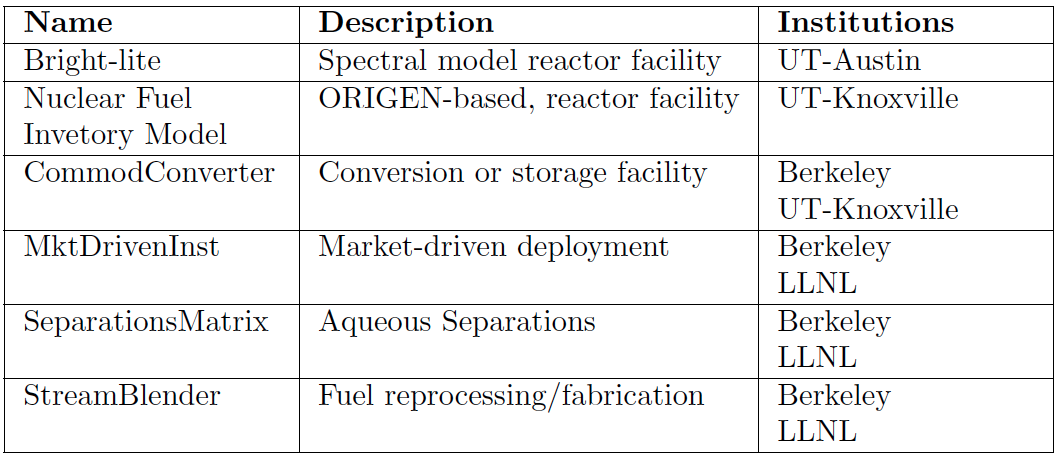## ...Well Beyond

### Algorithmic Sophistication

• Efficient: memory-efficient isotope tracking
• Customizable: constrained fuel supply
• Dynamic: isotopic-quality-based resource routing
• Physics-based: fuel fungibility

## Agent Based Systems Analysis

An agent-based simulation is made up of actors and communications between those actors.## Agent Based Systems Analysis

A facility might create material.## Agent Based Systems Analysis

It might request material.## Agent Based Systems Analysis

It might do both.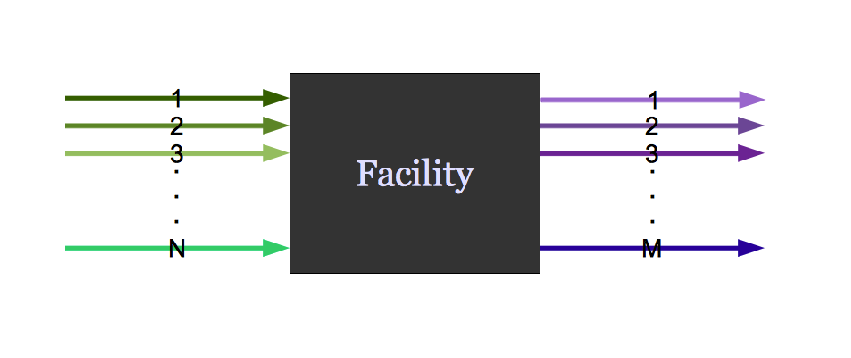## Agent Based Systems Analysis

Even simple fuel cycles have many independent agents.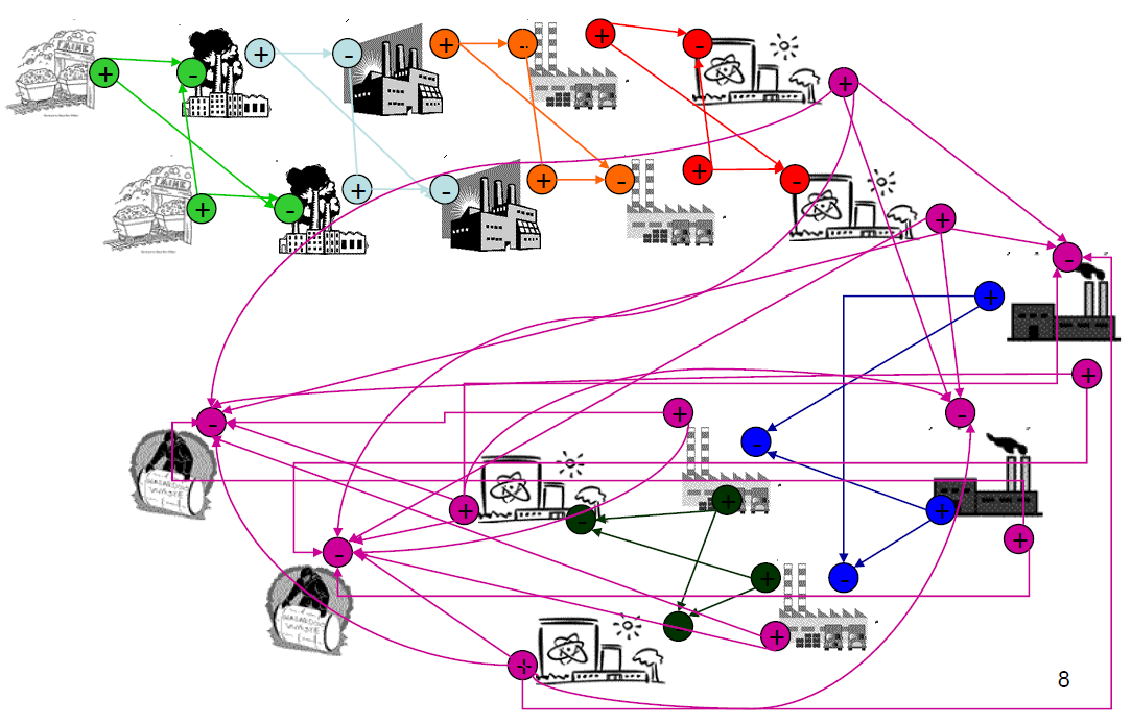## Dynamic Resource Exchange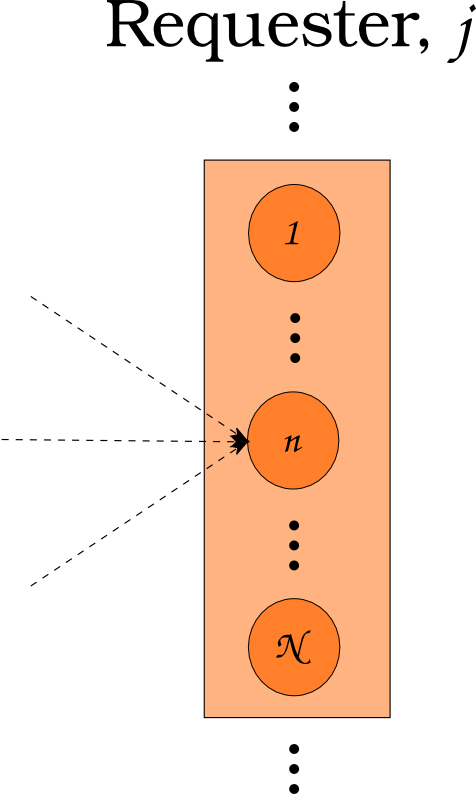$N_i \subset N$

## Dynamic Resource Exchange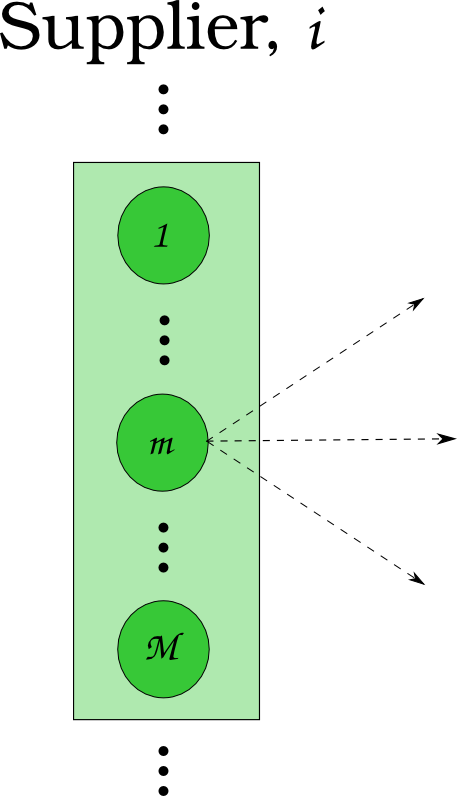$N_j \subset N$

## Dynamic Resource Exchange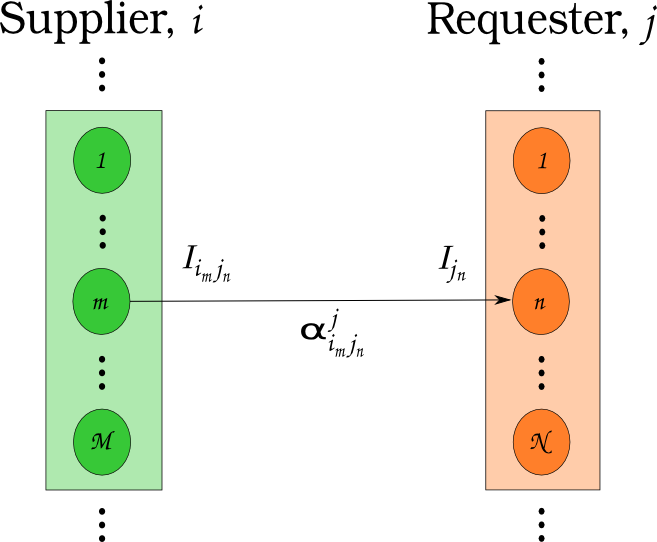$N_i \cup N_j = N$

## Feasibility vs. Optimization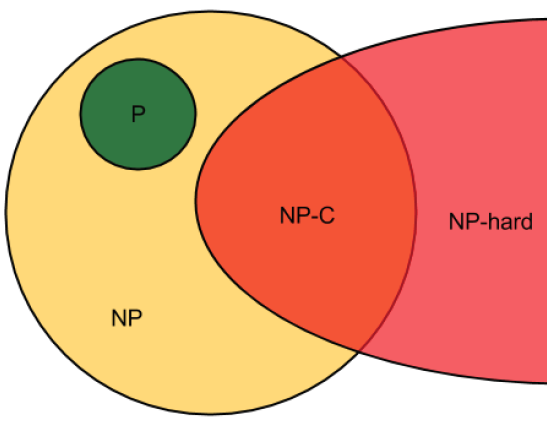If a decision problem is in NP-C, then the corresponding optimization problem is NP-hard.

## Multi-Commodity Transportation Formulation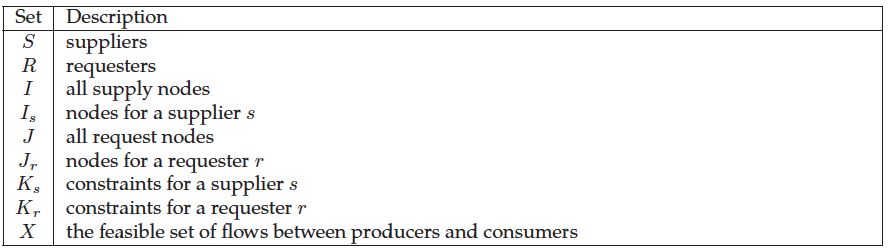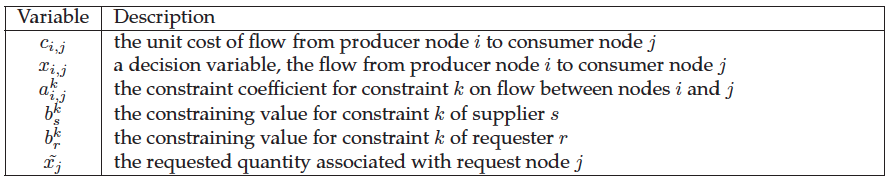## Multi-Commodity Transportation Formulation

\begin{align} \min_{x} z &= \sum_{i\in I}\sum_{j\in J} c_{i,j}x_{i,j} & \\ s.t & \sum_{i\in I_s}\sum_{j\in J} a_{i,j}^k x_{i,j} \le b_s^k & \forall k\in K_s, \forall s\in S\\ & \sum_{J\in J_r}\sum_{i\in I} a_{i,j}^k x_{i,j} \le b_r^k & \forall k\in K_r, \forall r\in R\\ & x_{i,j} \in [0,x_j] & \forall i\in I, \forall j\in J \end{align}

## Dynamic Resource Exchange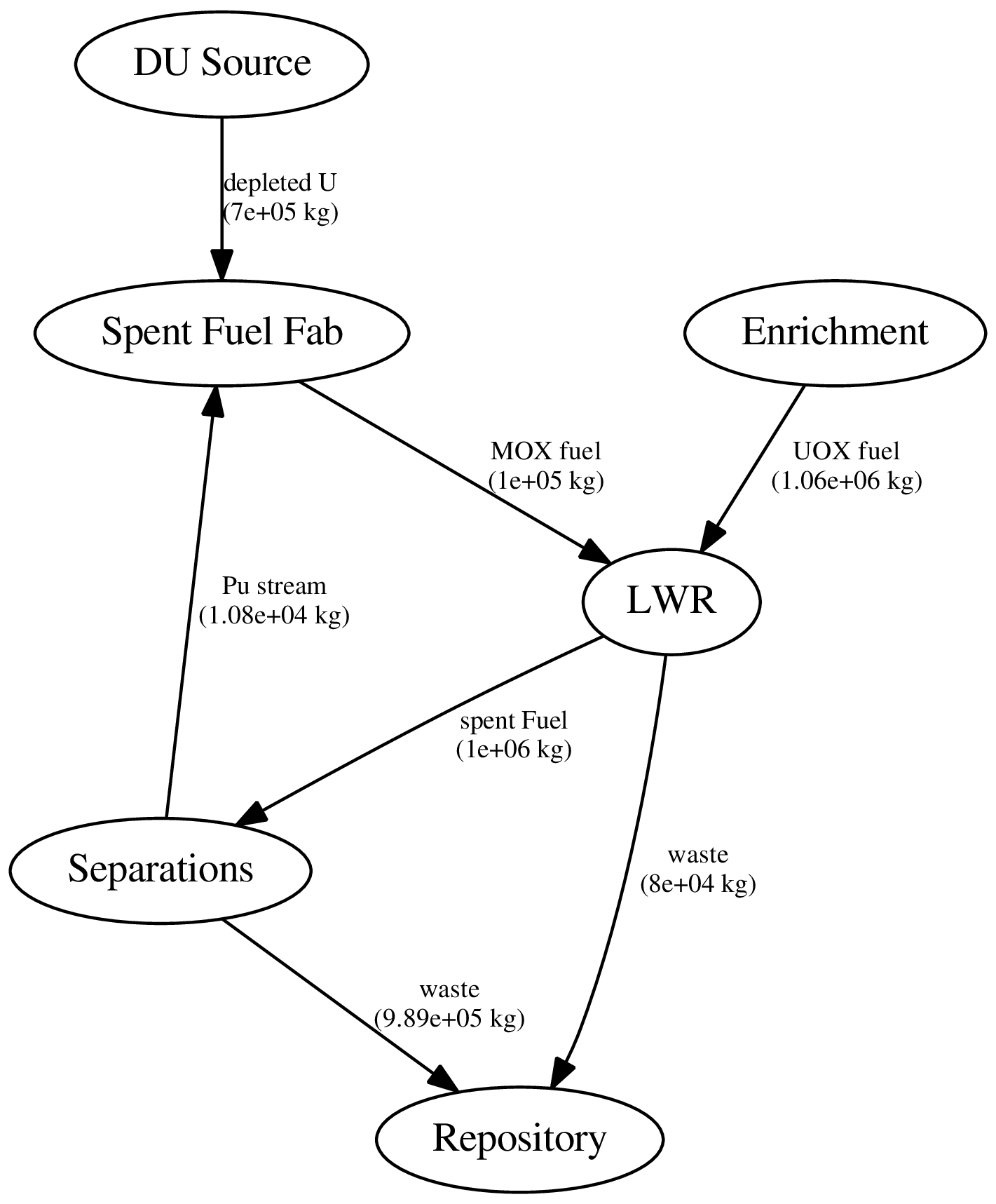## Dynamic Resource Exchange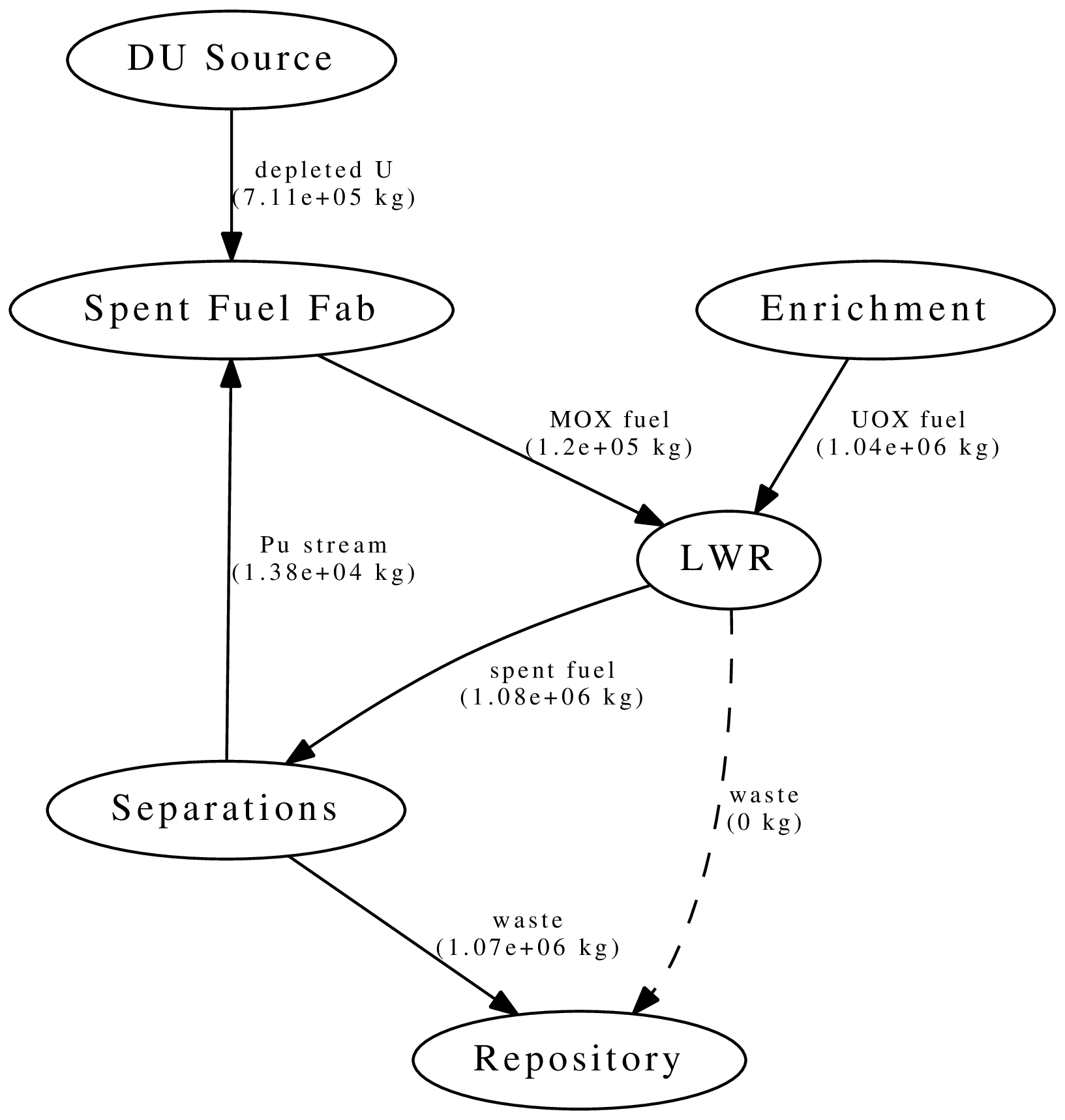## Dynamic Resource Exchange



mox
-    waste
+    spent_fuel
mox_fresh_fuel
mox_spent_fuel



## Dynamic Resource Exchange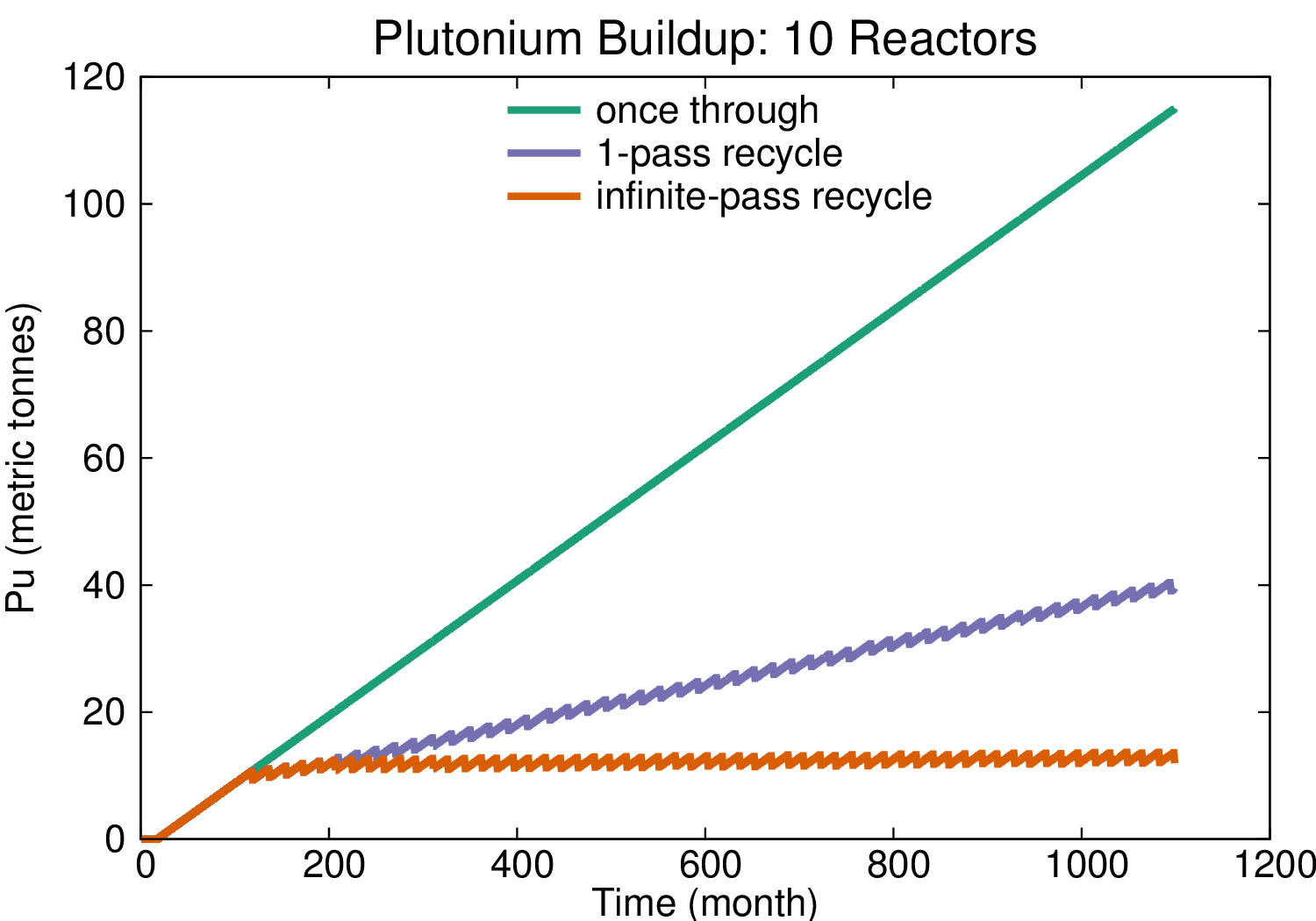## Transition Analysis

• LWR to SFR
• $T_0 = 2015$
• $T_f <= 2215$
• $C_0 = 100$ GWe LWR
• Annual nuclear energy demand growth: 1%
• Spent LWR fuel reprocessed to fabricate FR fuel
• Spent FR fuel reprocessed to fabricate FR fuel

## Transition Analysis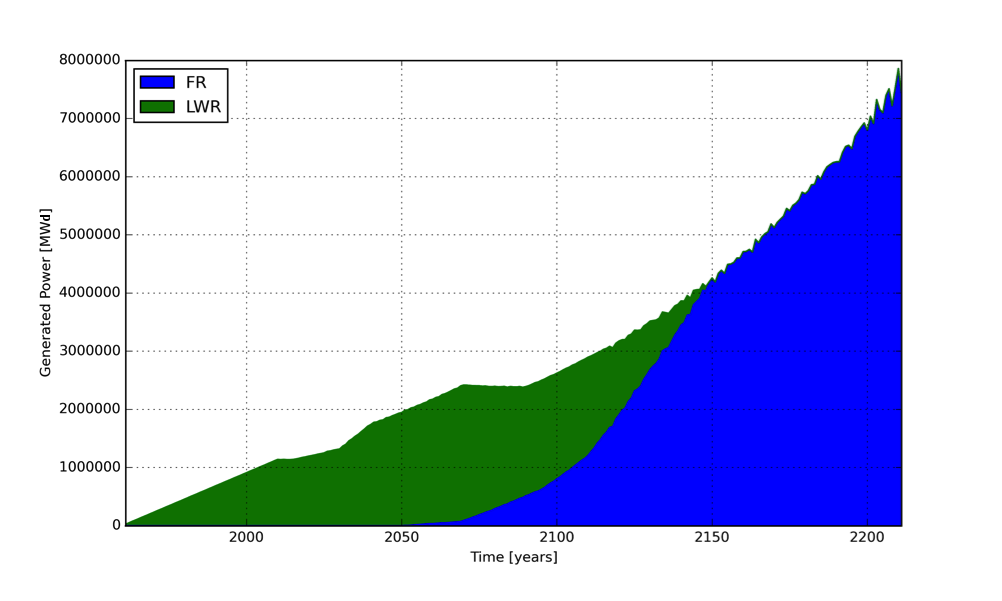Power generated by reactor type.

## Transition Analysis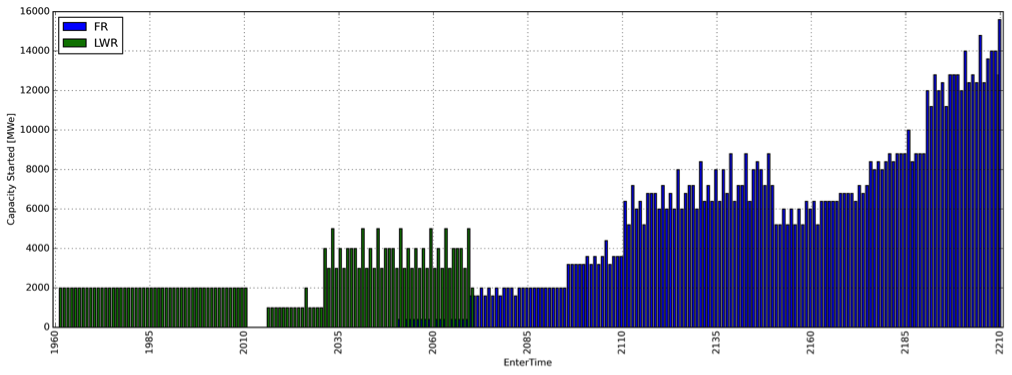Capacity deployed each year, by reactor type.

## How Do We Know It's Right?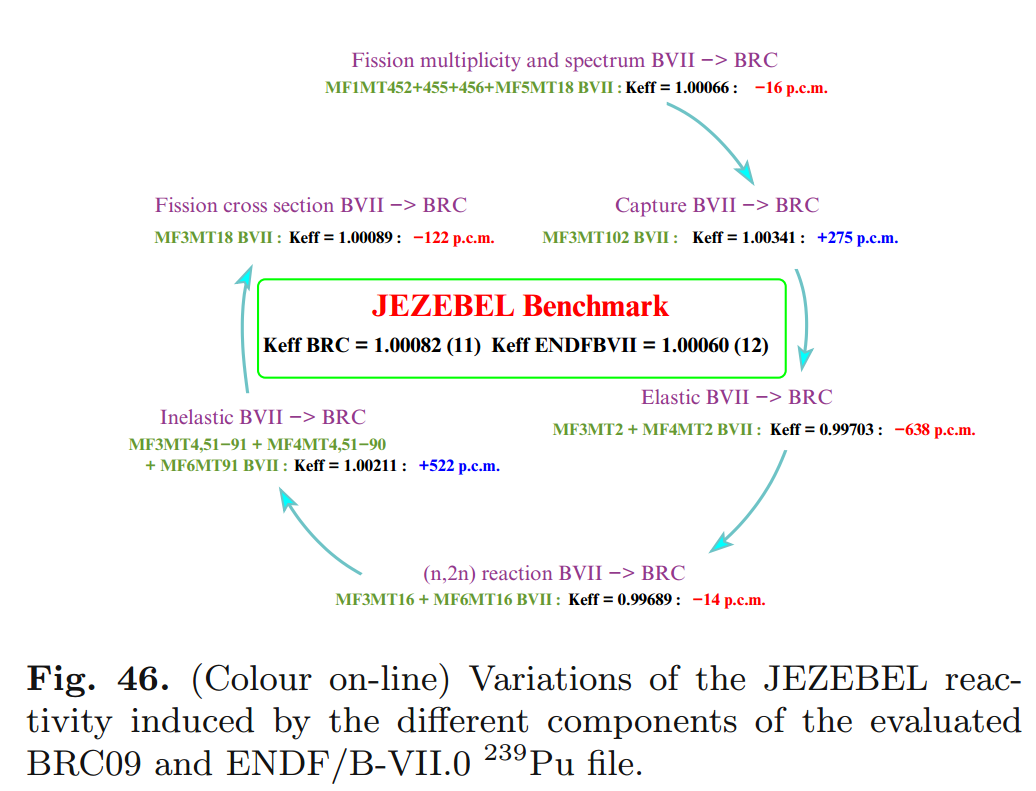## Quality Control

“ Organized Skepticism. Scientists are critical: All ideas must be tested and are subject to rigorous structured community scrutiny.” - R.K. Merton, 1942

## Unit Checking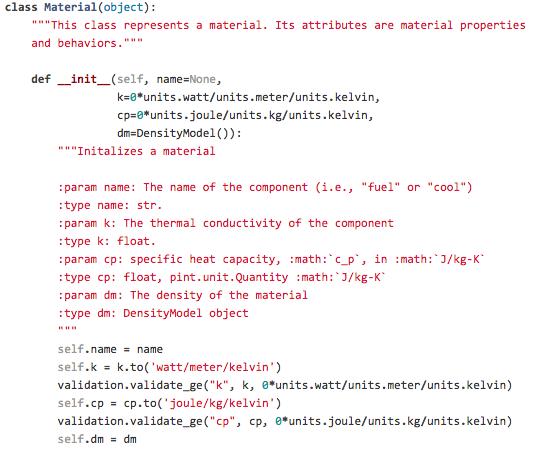In PyRK, the Pint package (pint.readthedocs.org/en/0.6/) is used keeping track of units, converting between them, and throwing errors when unit conversions are not sane.

## Backing Up Files

• Good: hope
• Better: nightly emails
• Best: remote version control

Version Control Systems: cvs, svn, hg, git

## Managing Changes

• Good: naming convention
• Better: clever naming convention
• Best: local version control

## Version Control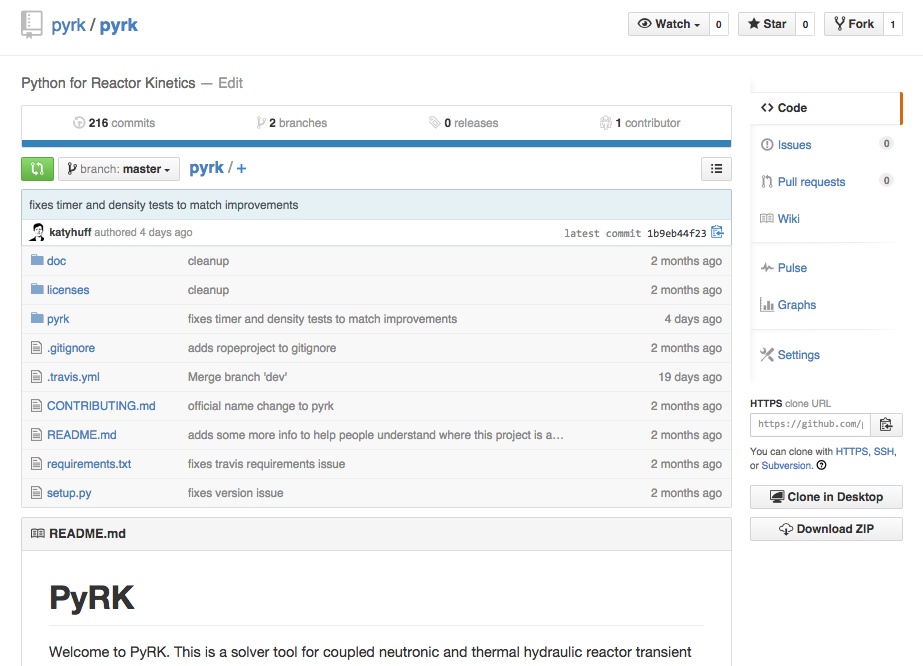Keeping track of versions of the code makes it possible to experiment without fear and placing the code online encourages use and collaboration.

## Automated Documentation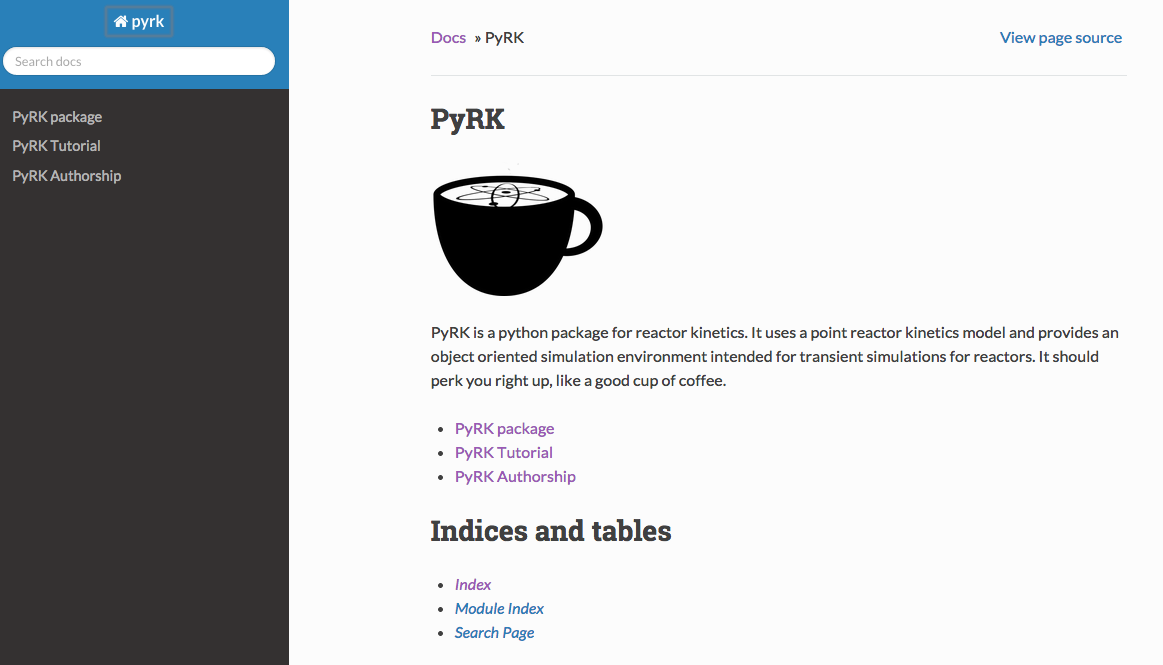Automated documentation creates a browsable website explaining the most recent version of the code.

## Error Detection

“ The scientific method’s central motivation is the ubiquity of error—the awareness that mistakes and self-delusion can creep in absolutely anywhere and that the scientist’s effort is primarily expended in recognizing and rooting out error. ” - Donoho, 2009.

## Error Detection

• Good: show results to experts
• Better: integration testing
• Best: unit test suite, continuous integration

## Test Suite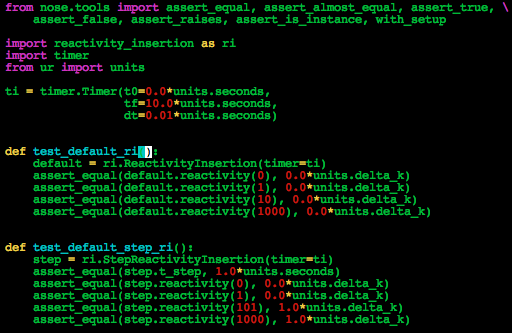The classes and functions that make up the code are tested individually for robustness using nose.

## Continuous Integration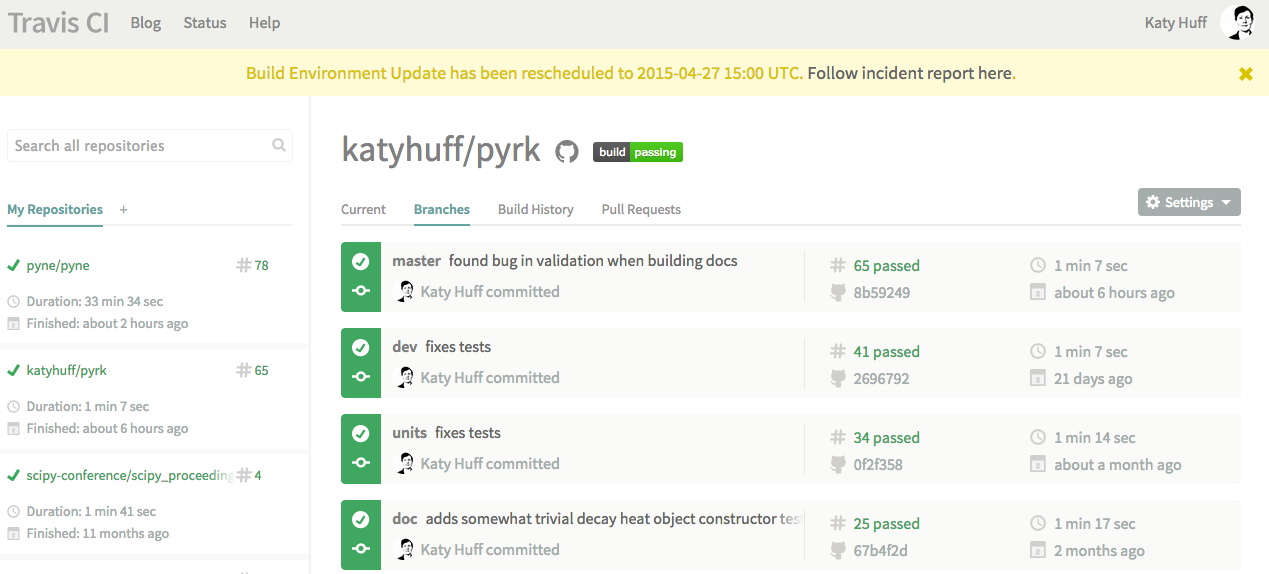The tests are run every time a change is made to the repository online. The results are public. If a main branch has a failed test, I get an email.

# THE END

### Katy Huff

katyhuff.github.io/2017-04-20-davisBased on a work at http://katyhuff.github.io/2017-04-20-davis.

## A Few of My Favorite Things

• C++, Python, Fortran
• xml, markdown, rst, $\LaTeX$
• Doxygen, sphinx
• CMake, conda, macports
• hdf5, sqlite
• cython, boost, Coin
• jekyll, reveal.js, beamer
• yt, matplotlib, paraview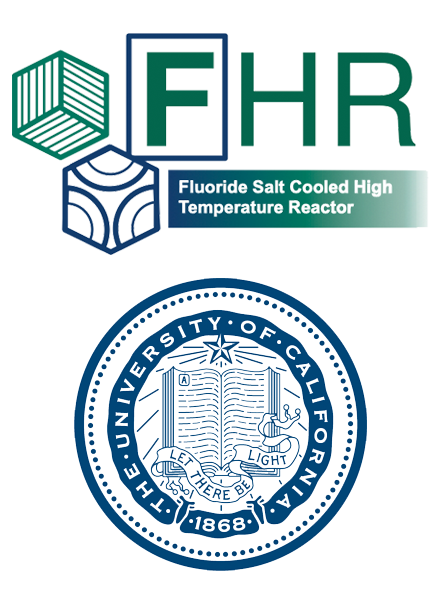## Transient Coupled Physics## Agent Based Systems Analysis

Hundreds of discrete facilities mine, mill, convert, fabricate, transmute, recycle, and store nuclear material.## Agent Based Systems Analysis

A facility might create material.## Agent Based Systems Analysis

It might request material.## Agent Based Systems Analysis

It might do both.## Agent Based Systems Analysis

Even simple fuel cycles have many independent agents.